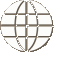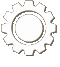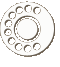COURSE Material
Course picture not available.
Foundations of Mathematics 30

Foundations of Mathematics 30 is divided into seven units. It meets the required outcomes outlined by Saskatchewan Learning. Students will require a TI-83 or TI-84 calculator in order to complete this course. This workbook series was developed for use by schools using an individualized, mastery-based learning system, and therefore comes with score keys, tests, and test keys. No additional text is necessary.

Unit 1: Financial Mathematics: Credit, Loans, and Tax

Upon completion of this unit, the student should be able to:

• calculate the monthly interest charges and service charges on an unpaid credit card
• identify and compare an instalment charge account and a thirty-day account
• calculate the monthly payments, total cost, and interest paid on a personal loan
• calculate mill rates, property taxes and discounts on taxes

Unit 2: Financial Mathematics: Compound Interest and Annuities

Upon completion of this unit, the student should be able to:

• understand the terminology used for compound interest and annuities
• calculate the future amount of a single deposit
• calculate the single present amount of a future amount
• calculate the interest rate required to produce a certain present or future amount
• use a program on a graphing calculator to determine a future amount, a present amount, or the interest rate
• calculate the future amount of an annuity
• calculate the equal payment amount to accumulate a certain future amount
• calculate the present amount of an annuity
• calculate the monthly payment required to pay off a loan

Unit 3: Permutations and Combinations

Upon completion of this unit, the student should be able to:

• apply the fundamental counting principle to determine the number of possibilities that exist in a given situation
• find the number of permutations of different objects
• find the number of permutations when only a portion of the set is taken
• find the number of permutations when objects are repeated
• understand the relationship between a permutation and the fundamental counting principle
• find the number of combinations of different objects from one set
• find the number of combinations of different objects from more than one set
• understand the difference between a permutation and a combination

Unit 4: Probability

Upon completion of this unit, the student should be able to:

• determine the probability that a single event will happen
• determine the probability that event A or event B will occur
• determine the probability that event A and event B will occur
• determine the probability that event A will occur given that event B has already occurred

Unit 5: Polynomial Functions

Upon completion of this unit, the student should be able to:

• divide polynomials
• find the remainder when a polynomial is divided by a binomial
• factor polynomials
• determine what quadrants the graphs of polynomial functions begin and finish in
• find the zeros of a polynomial function
• understand the meaning of the term zero of a polynomial function
• graph a polynomial function

Unit 6: Exponential and Logarithmic Functions

Upon completion of this unit, the student should be able to:

• understand what an exponential function is
• recognize the different types of exponential functions
• graph an exponential function
• shift the graph of an exponential function
• understand what a logarithm is
• evaluate logarithms
• change a logarithmic expression into an exponential expression and vice versa
• understand what a logarithmic function is
• recognize different types of logarithmic functions
• graph logarithmic functions

Unit 7: Sinusoidal Functions

Upon completion of this unit, the student should be able to:

• convert angle measurements from degrees to radians and vice versa
• graph the circular functions y = sin θ and y = cos θ
• determine the domain and range of the above functions
• graph the functions y = Asin Bx and y = Acos Bx
• graph the functions y = Asin B(x – C) + D and y = Acos B(x – C) + D
• determine the period length, the amplitude, the vertical shift, and the phase shift of the above graphs
• interpret equations of sinusoidal functions that apply to real life situations
COURSE MaterialsSAICS has developed Christian curriculum (where none other was available) to match Saskatchewan Learning objectives for high school courses.

View Our Course Listing
ORDERING CoursesFind out more information about ordering courses that have been developed by SAICS including score keys and associated textbooks.

Ordering Information